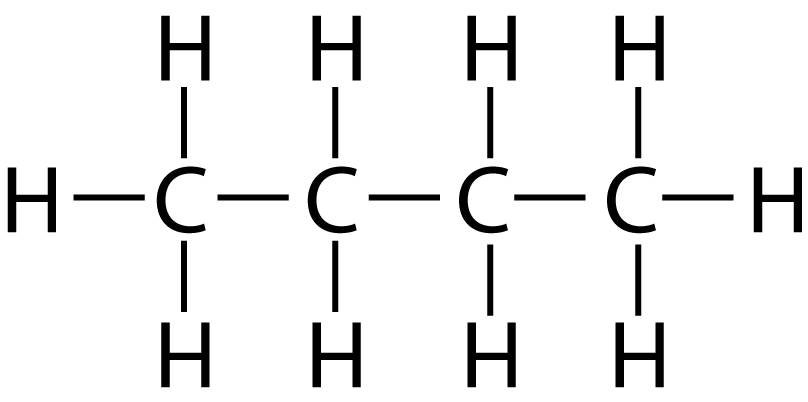Grab a calculator and a copy of the periodic table

(This test has only 10 questions, but may take 15 - 20 mins).

1. Calculate the relative formula mass (Mr) of calcium hydroxide, Ca(OH)2.
• A.   38
• B.   58
• C.   74
• D.   114
2. Which of the following contains the greatest number of moles?
• A.   2.4 g of carbon, C
• B.   2.3 g of sodium, Na
• C.   2.4 g of Magnesium, Mg
• D.   3.9 g of potassium, K
3. A student required 0.15 moles of magnesium oxide, MgO, for a reaction. What mass of MgO should she weigh out? [Mr of MgO is 40]
• A.   0.00375 g
• B.   0.0060 g
• C    6.0 g
• D.   267 g
4. Butane, a gas found in crude oil, has the structure shown here:What is the empirical formula of butane?

• A.   C4H10
• B.   C2H5
• C.   CH2
• D.   CH
5. 60.0g of calcium carbonate decomposed on heating to form calcium oxide and carbon dioxide.   The equation for the reaction is:

CaCO3  CaO + CO2

What is the maximum mass of calcium oxide which could be formed in this reaction?
[Mr values: CaCO3 = 100; CaO = 56; CO2 = 44]

• A.   26.4 g
• B.   30.0 g
• C.   33.6 g
• D.   56.0 g
6. An oxide of iron, on reduction, was found to contain 1.12 g of iron and 0.32 g of oxygen.

Calculate the empirical formula of this iron oxide.
[Relative atomic masses: Fe = 56; O=16]

• A: FeO
• B: Fe2O2
• C: Fe2O
• D: Fe2O3

7. A carbohydrate was found to have an empirical formula CH2O and a relative molecular mass of 120. The molecular formula of the carbohydrate is …

• A.   CH2O
• B.   C2H4O2
• C.   C4H8O4
• D.   C6H12O6
8. 1.38 g of sodium was burned in oxygen. An oxide of mass 2.34 g was formed.

Calculate the moles of sodium and oxygen atoms in the final product.
[Ar of Na = 23; Ar of O = 16]

 Moles of sodium atoms Moles of oxygen atoms A 0.06 0.146 B 0.06 0.06 C 0.102 0.06 D 0.102 0.146

Q9-10: 34.0 kg of ammonia, NH3 is reacted with excess nitric acid, HNO3, to produce ammonium nitrate fertilizer. The equation for the reaction is:

NH3  + HNO3  NH4NO39. Calculate the number of moles of ammonia, NH3, used in this reaction.

[Relative atomic masses H = 1; N = 14, O = 16]

• A.   2.27
• B.   2.00
• C.   2267
• D.   2000
10. Calculate the maximum mass, in kg, of ammonium nitrate formed [Mr of NH4NO3 is 80]
• A.   181.6 kg
• B.   160 kg
• C.   181,600 kg
• D.   160,000 kg